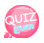# function 2

ASCII code conversion function: : ord() chr()

To convert a string to ASCII code, use ord(). To convert ASCII code to a string, use chr().
ASCII code value of uppercase alphabe A ~ Z is 65 ~ 90 Lowercase letters a-z are 97-122.

```<?php
echo "Alphabetical output using ASCII code chr()<br />";
echo "Alphabet capital letter A-Z output<br />";

for(\$a = 65; \$a <= 90; \$a++){
echo chr(\$a);
echo " ";
}
echo "<br />";
echo "Lowercase alphabet a-z output <br />";

for(\$a = 97; \$a <= 122; \$a++){
echo chr(\$a)." ";
}
?>```The capital letter A is ASCII code 65.
In other words, it is A by converting 65 to chr().
String output functions echo(), print()
echo(), print()
String tags, etc. display double quotes.
String = "hello"
Tag = <br /> Example of use)
echo "Hello.";
echo "<br />";
Formatted output functions printf(), sprintf() functions
It's the way it comes from the language.
printf(type specifier, variable1, variable2, ... variable n)
sprintf(type specifier, variable1, variable2, ... variable n)The following example outputs a formatted calculation.

```<?php
echo "Formatted calculations using the print() function <br />";

\$a = 97.458;
\$b = 95.956;

\$ab = \$a * \$b;

\$form1 = sprintf("\ %0.2f", \$a*\$b); // The product of a and b is output and is expressed to two decimal places.
\$form2 = sprintf("\ %0.3f", \$a/\$b); // The result of dividing a and b is displayed and is expressed to the third decimal place.

echo "When the variable is a = \$a, b = \$b<br />";

printf("1.The result of the addition of% 0.3f and% 0.3f ...[%0.2f]", \$a, \$b, \$a+\$b);
echo "<br />";
printf("1.Subtraction of% 0.3f and% 0.3f ...[%0.2f]", \$a, \$b, \$a-\$b);
echo "<br />";
echo "3. The result of multiplying \$a and \$b ....{\$form1} <br />";
echo "4. The result of division of \$a and \$b ...{\$form2} <br />";
?>```printf(% 0.2f <--- This is the place to put the value of the variable, and 0.2f is the second decimal place. F is a real number. Then, you declare the variable to be entered.

Here's the easy source:

```<?php
\$a = 10;
\$b = 20;

printf(" %u + %u = %u", \$a, \$b, \$a+\$b);
?>```

결과
10 + 20 = 30